###Everything you need to master calculus!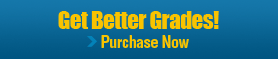What is the Calculus Study Guide?
The Calculus Study Guide is an electronic book intended to help a student taking a first course in calculus.

Comprehensive Course Materials
• Covers all topics in an introductory calculus course, and includes more than 150 examples
• 17 interactive Maple tutors to help you understand the concepts
• Overviews of each topic, hyperlinked definitions, glosseries and index.
Test Your Knowledge and Practice
• Over 100 Maple-graded questions
• Free-form entry of responses, instant feedback, and hints
• Questions contain randomly generated components, so they are different each time

### Screenshots and Examples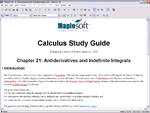Chapter Introduction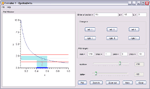Exclusive Maplet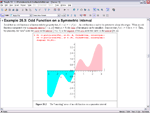Example Solution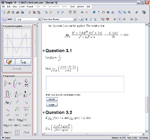Maple-graded question

### The 31 chapters are divided into five units as follows:

Limits

1. Conceptual Understanding of the Limit
2. Precise Definition of the Limit
3. Limit Laws
4. Limits of Trigonometric Functions
5. Limits at Infinity
6. Infinite Limits
7. Continuity
8. Intermediate Value TheoremDerivatives

9. Conceptual Understanding of the Derivative
10. Precise Definition of the Derivative
11. Derivative Rules
12. Chain Rule
13. Derivatives of Trigonometric Functions
14. Implicit DifferentiationApplications of Derivatives

15. Related Rates
16. Global Analysis
17. Local Analysis
18. Graphing
19. Optimization
20. Mean Value Theorem
Integrals

21. Indefinite Integrals (Antiderivatives)
22. Differential Equations
23. Riemann Sums
24. Definite Integrals
25. Fundamental Theorems of Calculus
26. Evaluating Definite Integrals with the Fundamental TheoremsDerivatives

27. Area of a Plane Region
28. Mean Value Theorem for Integrals
29. Volume of a Solid
30. Length of a Curve
31. Work